# 从纹理中生成法线贴图

711501594

## 高度图或灰度图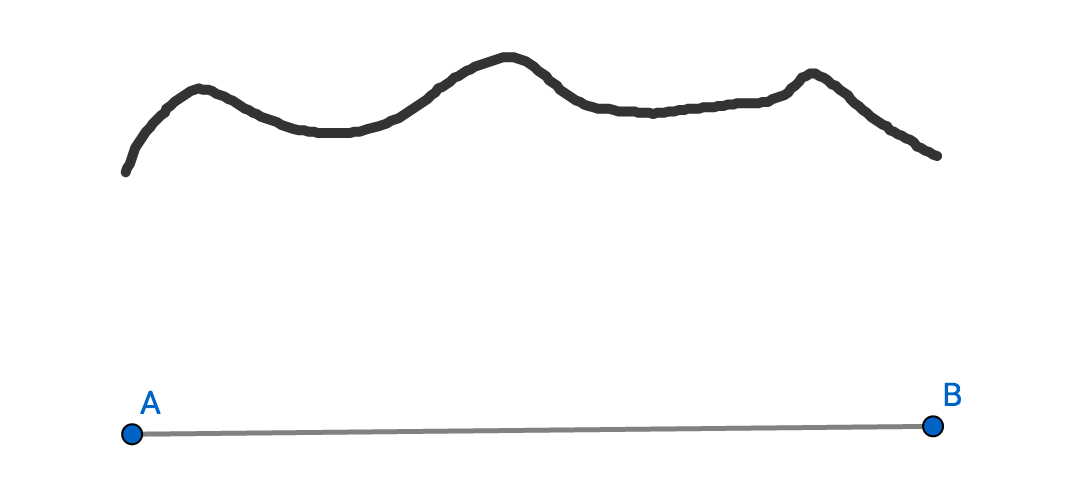`color.r * 0.2126 + color.g * 0.7152 + color.b * 0.0722`

## 计算方法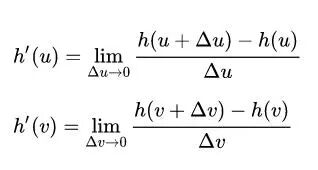和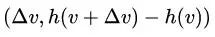。如果你觉得乱，没关系，后面看具体的代码就明白了。

## 实例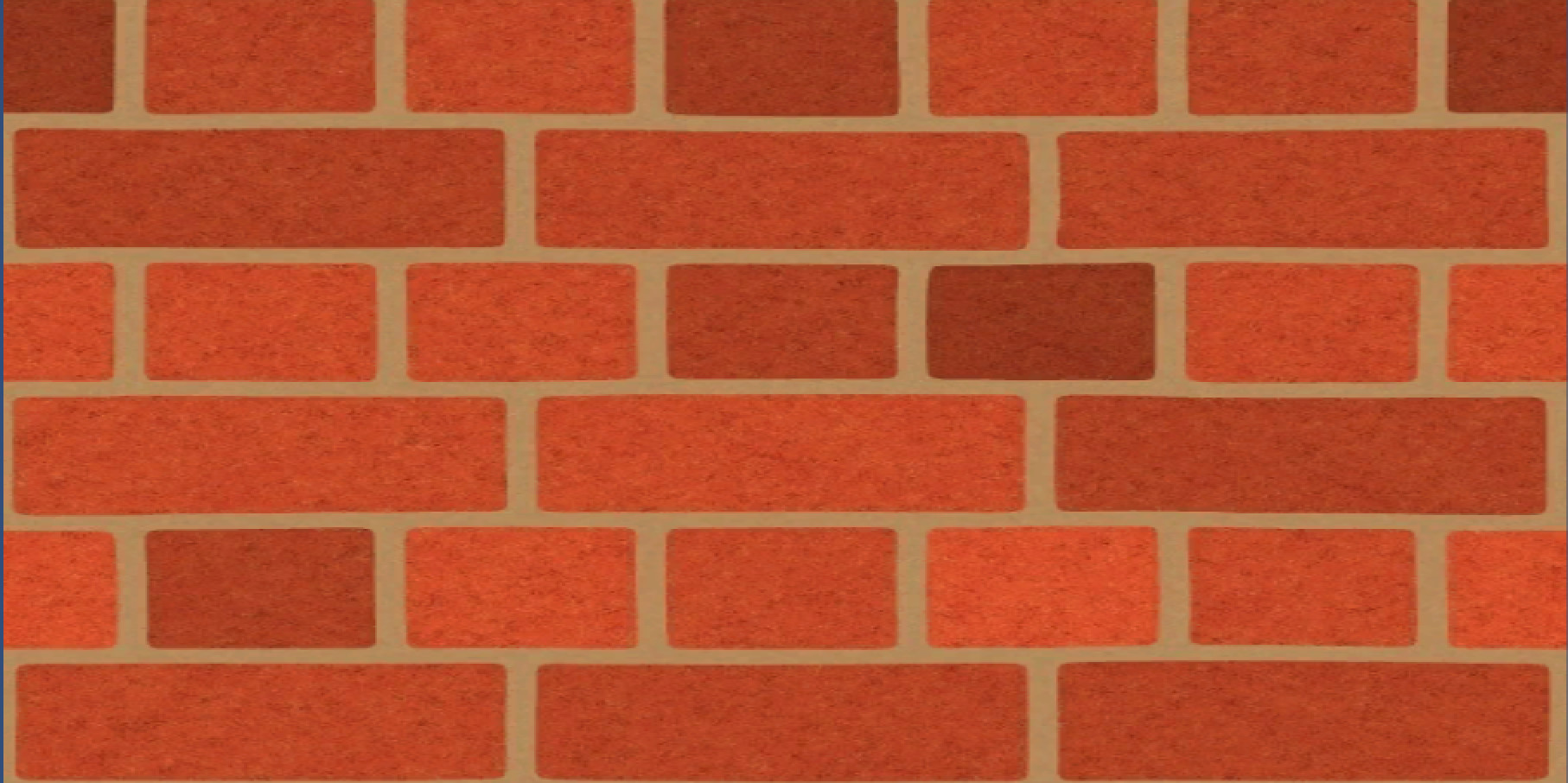```float GetGrayColor(float3 color)｛
return color.r * 0.2126 + color.g * 0.7152 + color.b * 0.0722;｝```

```float3 GetNormalByGray(float2 uv)｛
// 代码后有详细的讲解
float2 deltaU = float2(_MainTex_TexelSize.x * _DeltaScale, 0);
float h1_u = GetGrayColor(tex2D(_MainTex, uv - deltaU).rgb);
float h2_u = GetGrayColor(tex2D(_MainTex, uv + deltaU).rgb);
// float3 tangent_u = float3(1, 0, (h2_u - h1_u) / deltaU.x);
float3 tangent_u = float3(deltaU.x, 0, (h2_u - h1_u));
float2 deltaV = float2(0, _MainTex_TexelSize.y * _DeltaScale);
float h1_v = GetGrayColor(tex2D(_MainTex, uv - deltaV).rgb);
float h2_v = GetGrayColor(tex2D(_MainTex, uv + deltaV).rgb);
// float3 tangent_v = float3(0, 1, (h2_v - h1_v) / deltaV.y);
float3 tangent_v = float3(0, deltaV.y, (h2_v - h1_v));
float3 normal = normalize(cross(tangent_v, tangent_u));
return normal;｝```

`fixed4 color = normal * 0.5 + 0.5`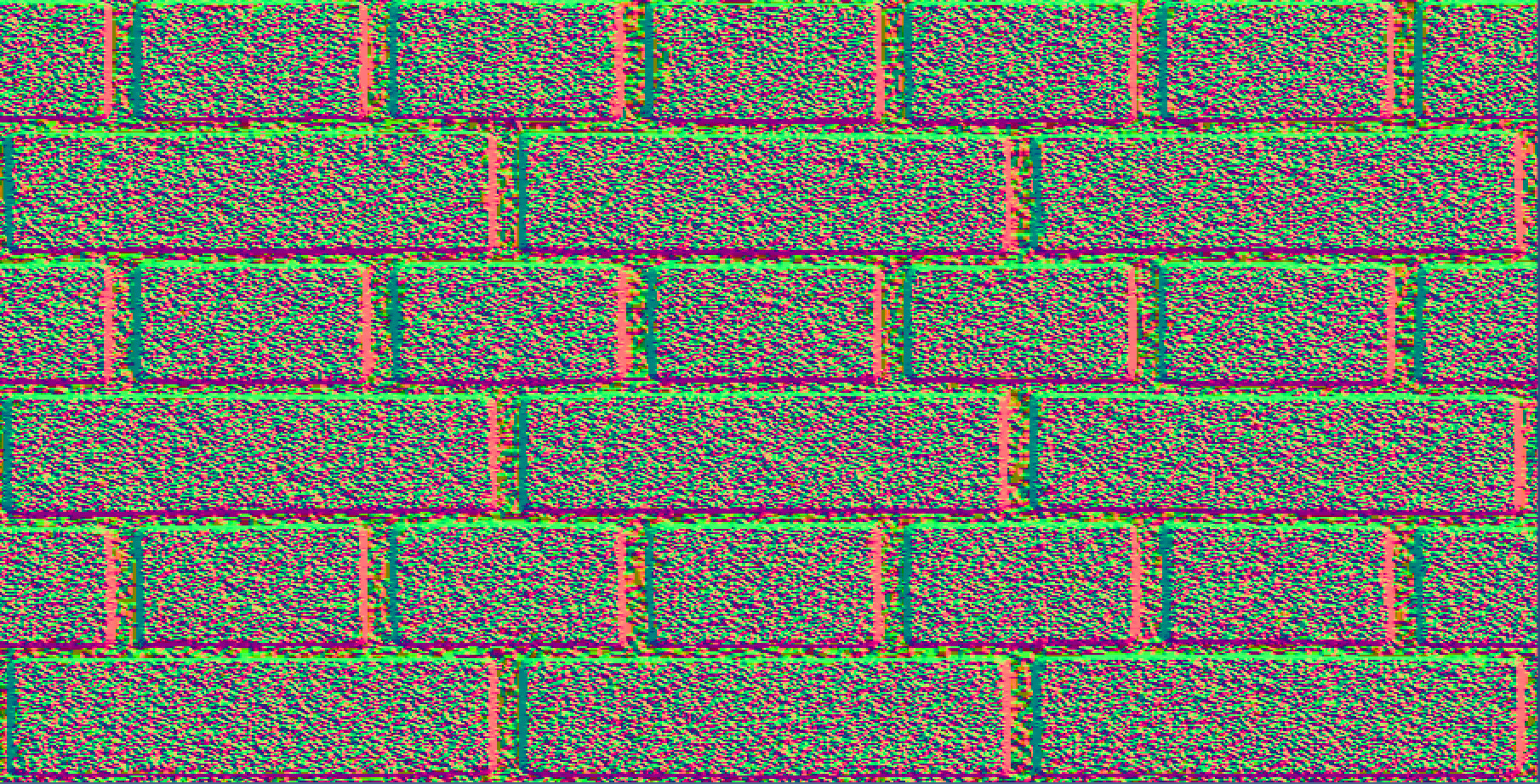`normal.z *= -1;fixed4 color = normal * 0.5 + 0.5`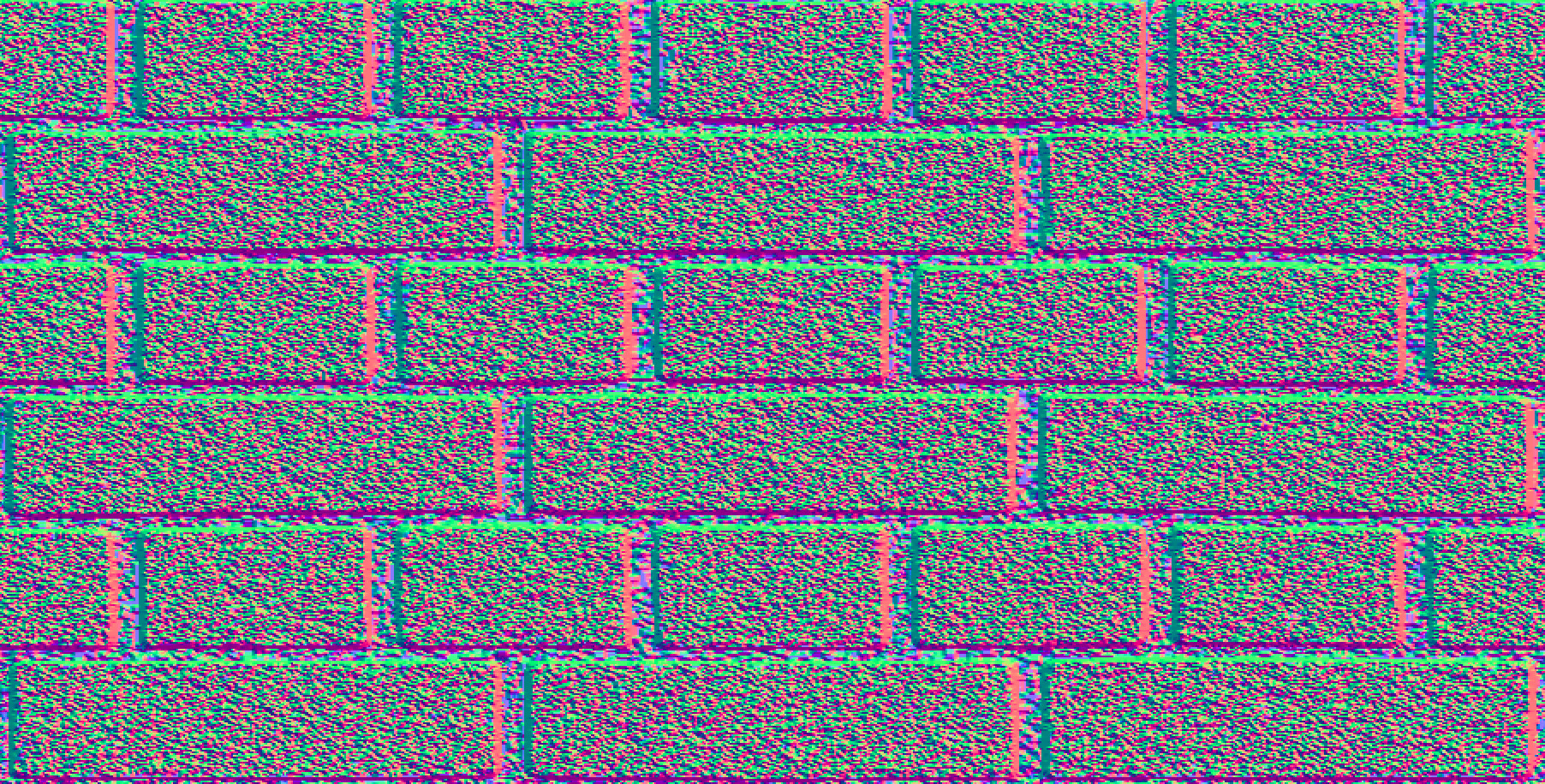```float3 GetNormalByGray(float2 uv)｛
...
float3 tangent_u = float3(deltaU.x, 0, _HeightScale * (h2_u - h1_u));
...
float3 tangent_v = float3(0, deltaV.y, _HeightScale * (h2_v - h1_v));
...｝```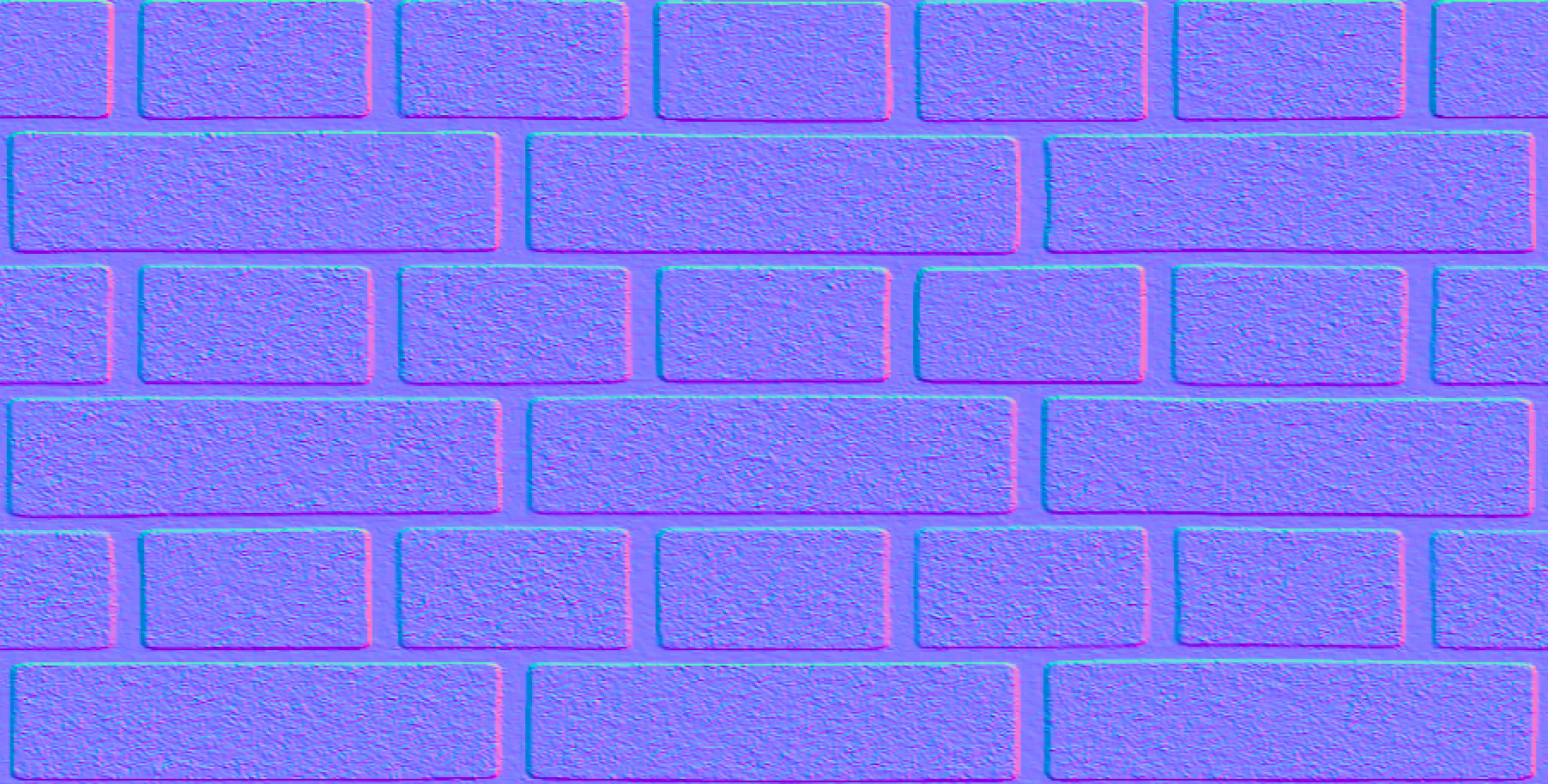## 加上光照

```fixed4 frag (v2f i) : SV_Target｛
float3 normal = float3(0, 0, -1);
fixed4 texColor = tex2D(_MainTex, i.uv);
float diffuse = saturate(dot(normal, normalize(_WorldSpaceLightPos0.xyz)));
fixed4 color;
color.rgb = texColor.rgb * diffuse *_LightColor0.rgb;
return color;｝```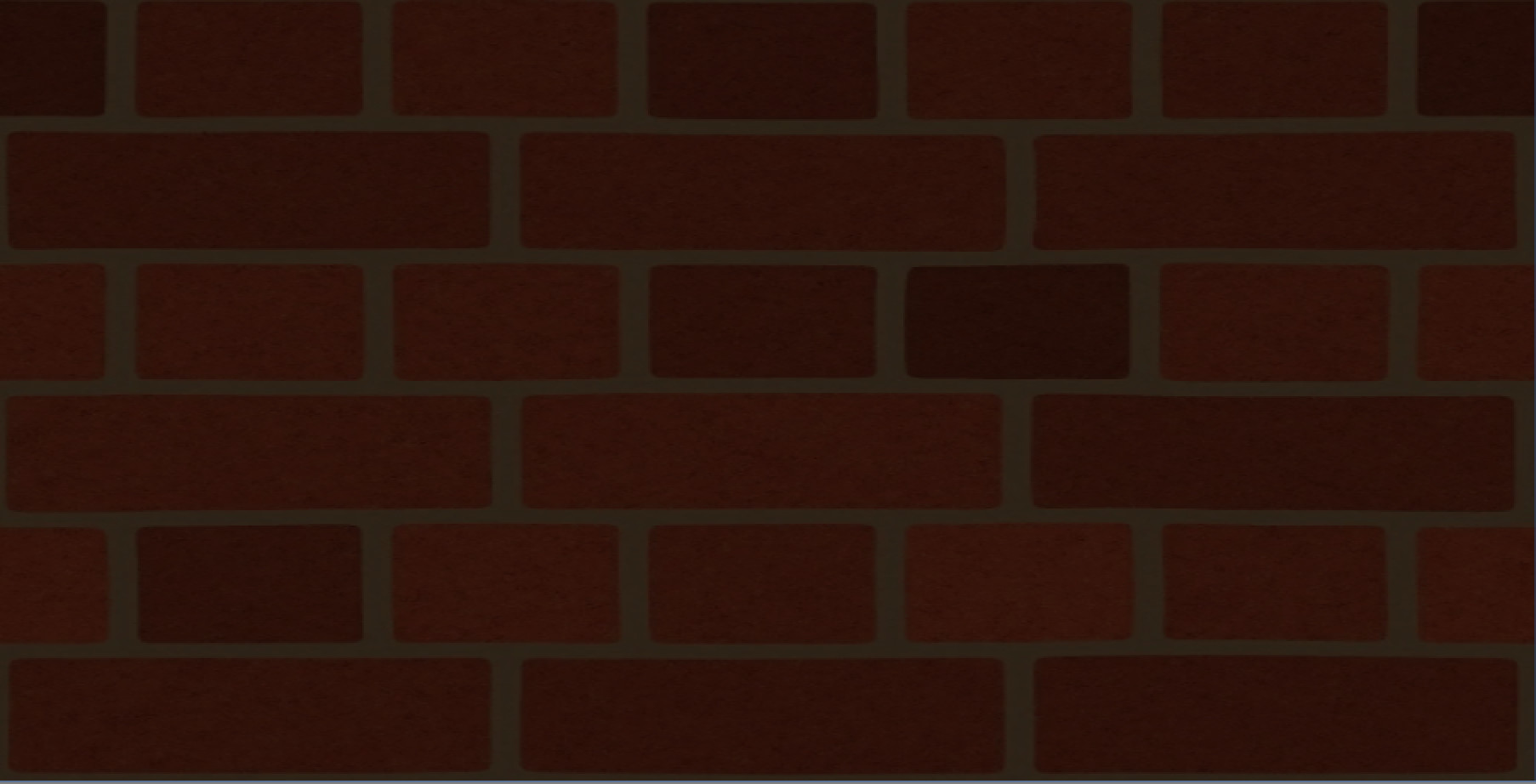```fixed4 frag (v2f i) : SV_Target｛
float3 normal = GetNormalByGray(i.uv);
// normal.z *= -1;
fixed4 color;
fixed4 texColor = tex2D(_MainTex, i.uv);
float diffuse = saturate(dot(normal, normalize(_WorldSpaceLightPos0.xyz)));
color.rgb = texColor.rgb * diffuse *_LightColor0.rgb;
return color;｝```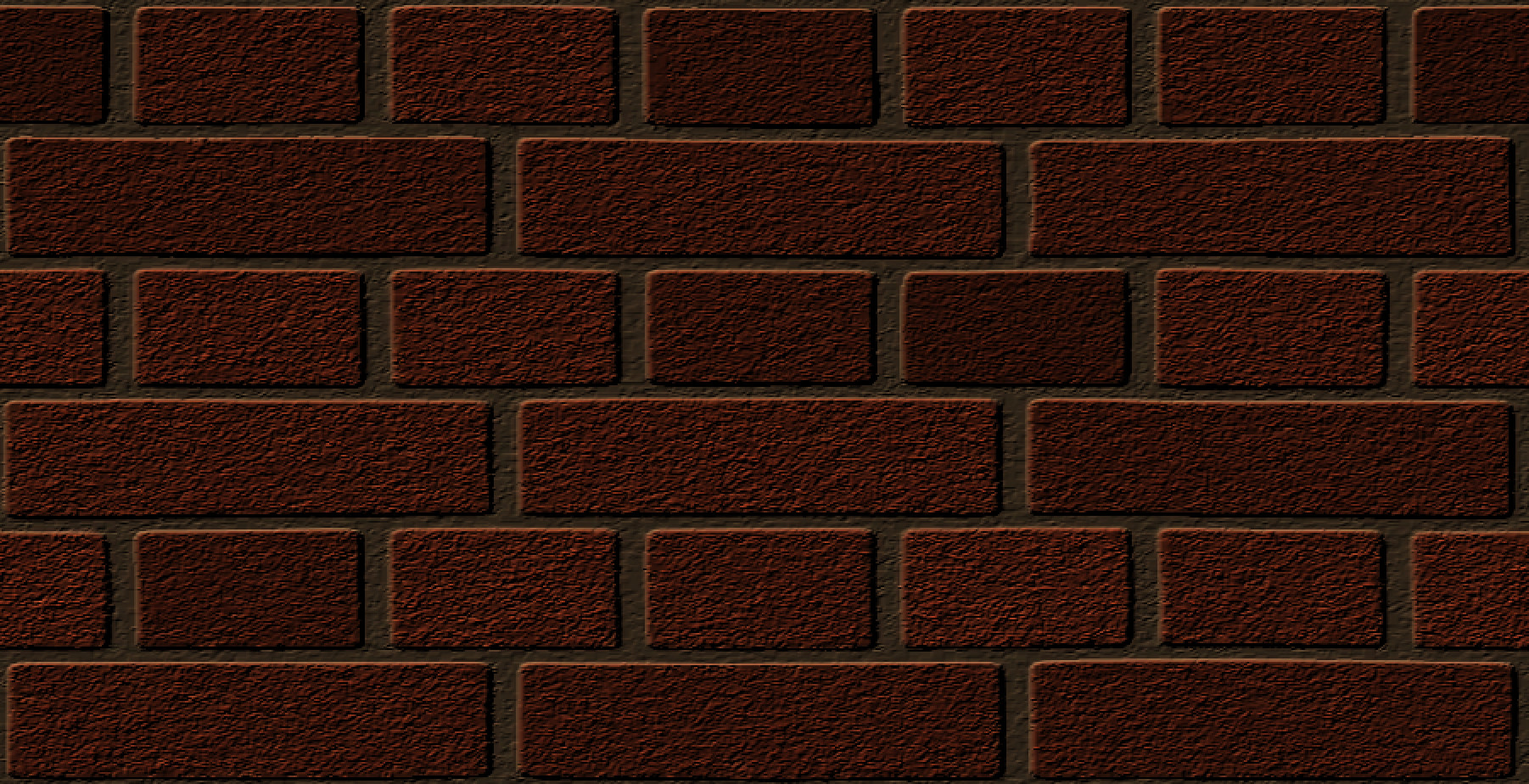## 最后的工作